# Insert 5

Insert five harmonic means between 1/2 and 1/26

a2 =  0.1667
a3 =  0.1
a4 =  0.0714
a5 =  0.0556
a6 =  0.0455

### Step-by-step explanation:Did you find an error or inaccuracy? Feel free to write us. Thank you!Tips to related online calculators
Looking for calculator of harmonic mean?
Looking for a statistical calculator?

## Related math problems and questions:

• Insert 6Insert four harmonic means between 3/7 and 3/19
• Insert 7Insert five harmonic means between 3 and 18
• Harmonic mean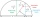If x, y, z form a harmonic progression, then y is the harmonic mean of x and z. Find the harmonic mean of the numbers 6 and 5.
• HP - harmonic progressionDetermine the 10th term of the harmonic progression 6,4,3,…
• Harmonic meanHarmonic means of 6 and 12
• Harmonic seriesInsert four members between 5/3 and 5/11 to form harmonic series (means).
• HP - harmonic progressionDetermine the 8th term of the harmonic progression 2, 4/3, 1,…
• Harmonic and arithmetic means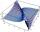The local Utah Department of Child Service office wants to project staffing needs based on current social worker assignments. They have the number of cases per social worker for the following staff: Mary: 25 John: 35 Ted: 15 Lisa: 45 Anna: 20 Calculate: a
• Insert AP memberInsert arithmetic means between 75 and 180.
• Insert 4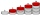Insert three arithmetic means between 3 and 63.
• Sequence 11What is the nth term of this sequence 1, 1/2, 1/3, 1/4, 1/5?
• Harmonic 4The harmonic mean of -6 and 5.
• HP - harmonic progression 2Compute the 16th term of the HP if the 6th and 11th term of the harmonic progression are 10 and 18 respectively.
• Insert 3Insert five arithmetic progression members between -7 and 3/2.
• Insert into GPBetween numbers 5 and 640, insert as many numbers to form geometric progression so the sum of the numbers you entered will be 630. How many numbers must you insert?
• Cyclist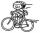The cyclist goes uphill 10 km for 50 minutes and downhill minutes for 29 minutes, both applied to the pedals the same force. How long he pass 10 km by plane?
• Cleaners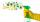Milan would clean up the room for 2.5 hours, Eric would take 10 hours. How long they swept the room together?